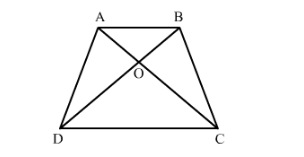# ABCD is a trapezium in which AB || CD. The diagonals AC and BD intersect at O. Prove that :

Question:

ABCD is a trapezium in which AB || CD. The diagonals AC and BD intersect at O. Prove that :(i) $\triangle \mathrm{AOB} \sim \Delta \mathrm{COD}$ (ii) If $\mathrm{OA}=6 \mathrm{~cm}, \mathrm{OC}=8 \mathrm{~cm}$,

Find:

(a) Area ∆AOBArea ∆COD
(b) Area ∆AODArea ∆COD

Solution:

Given: ABCD is a trapezium in which AB || CD.

The diagonals AC and BD intersect at O.

To prove:

(i) $\triangle \mathrm{AOB} \sim \Delta \mathrm{COD}$

(ii) If $\mathrm{OA}=6 \mathrm{~cm}, \mathrm{OC}=8 \mathrm{~cm}$

To find:

(a) $\frac{\operatorname{ar}(\Delta \mathrm{AOB})}{\operatorname{ar}(\Delta \mathrm{COD})}$

(b) $\frac{\operatorname{ar}(\Delta \mathrm{AOD})}{\operatorname{ar}(\Delta \mathrm{COD})}$

Construction: Draw a line MN passing through O and parallel to AB and CD

(i) Now in ΔAOB and ΔCOD
∠OAB=∠OCD        Alternate angles∠OBA=∠ODC        Alternate angles

$\angle \mathrm{AOB}=\angle \mathrm{COD}($ vertically opposite angle $)$

$\Rightarrow \Delta \mathrm{AOB} \sim \Delta \mathrm{COD}$ (A.A.Acrieteria)(ii) (a)We know that the ratio of areas of two similar triangles is equal to the ratio of squares of their corresponding sides.

$\frac{a r(\Delta \mathrm{AOB})}{a r(\Delta \mathrm{COD})}=\left(\frac{\mathrm{OA}}{\mathrm{OC}}\right)^{2}$

$=\left(\frac{6}{8}\right)^{2}$

$\frac{a r(\Delta \mathrm{AOB})}{a r^{\prime}(\Delta \mathrm{COD})}=\frac{9}{16}$

(b)We know that the ratio of areas of two similar triangles is equal to the ratio of squares of their corresponding sides.

ar∆AODar∆COD=OAOC2                     =6cm8cm2                     =916